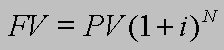## Friday, June 6, 2008

### Investing at 15% per yearCase C: Investing \$1000 at 15% per year

Suppose you invest \$1000 today at a 15% rate of return. Using the equation above, we can calculate the future value of this one time investment.

FV = (\$1,000) (1.15)n where n = the number of years

After 10 years, this single \$1,000 investment will be worth:

FV = (\$1,000) (1.15)10 = \$4,046

After 20 years, this single \$1,000 investment will be worth:

FV = (\$1,000) (1.15)20 = \$16,367

After 30 years, this single \$1,000 investment will be worth:

FV = (\$1,000) (1.15)30 = \$66,212

After 40 years, this single \$1,000 investment will be worth:

FV = (\$1,000) (1.15)40 = \$267,864

After 50 years, this single \$1,000 investment will be worth:

FV = (\$1,000) (1.15)50 = \$1,083,657

A single investment of \$1,000 that earns a 15% rate of return for 40 years turns into over a quarter of a million dollars. If it is invested for 50 years, it becomes more than a million dollars!

#### 1 comment:

1.At 15% money doubles every five years so a business that grows at 15% per year would double in size every five years.